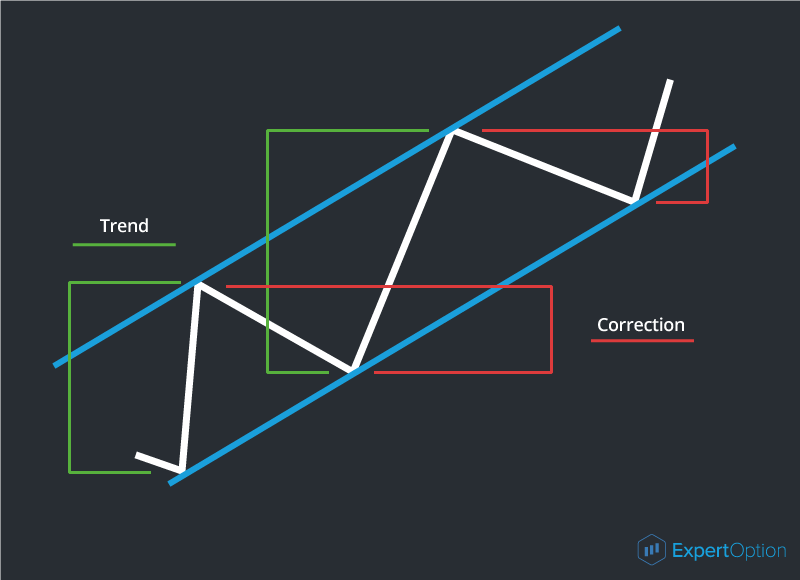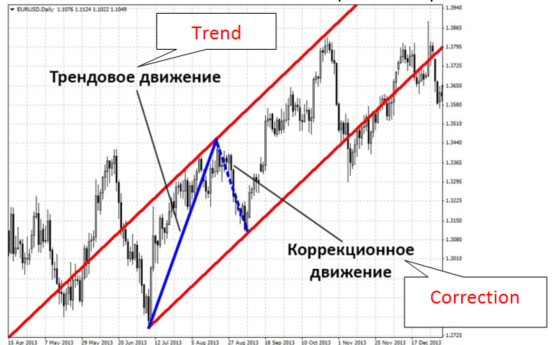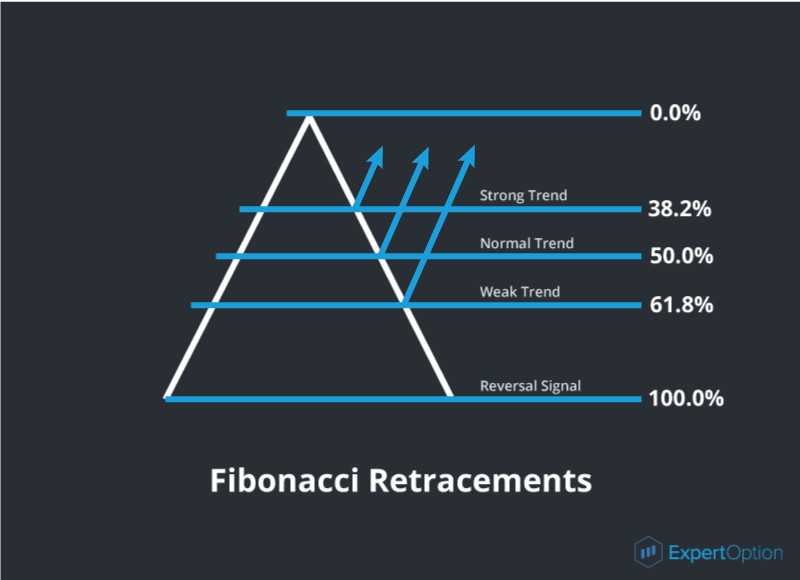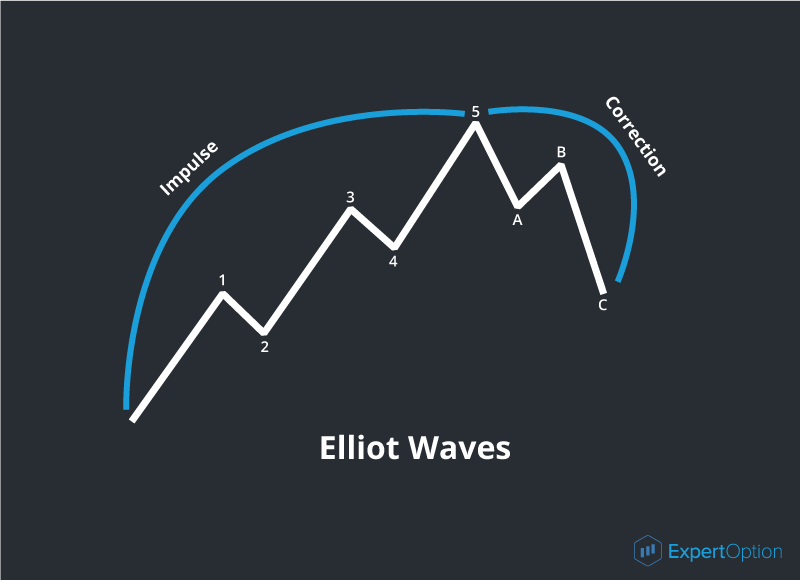Home

# Fibonacci Retracements and Elliot Waves

When a tendency is reversing or a new tendency is forming we always ask a question: “How much will the price move?” The different methods such as Fibonacci retracements help us to answer this question.

At first let us understand what a retracement is. A retracement (correction) is a movement in the trend channel against to its direction. Thanks to the correction a trend channel is formed. You can see the example on the picture:Any movement is followed by the correction. And this is a structure of the market: at the beginning of the movement all participants bought and when the price movement stopped they wished to fix their profits and started to sell. This is a reason of the correction. The correction strength depends on a number of participants in the market.

And here we have a question “How much deep will the correction be?” The Fibonacci retracements will help us to answer it. The Fibonacci Retracements are the horizontal levels used to suggest the target correction length. Let us see how it works. Leonardo Fibonacci was a famous Italian philosopher, mathematician and trader who promoted appearance of Arabic numerals in Europe. The other his achievement was a sequence of numbers later known as Fibonacci numbers. In the Fibonacci sequence of numbers, each number is the sum of the previous two numbers: 0, 1, 1, 2, 3, 5, 8, 13, 21, 34, 55, 89 etc. A legend tells that he discovered his sequence of numbers while watching the breeding of rabbits. This sequence cannot help us in the market but its properties give us the useful ratios which we meet in life and which correspond to the golden ratio rule. Here are the useful properties we need:

1) Dividing one number in the series by the number that follows gives us the ratio of 0,618

2) Dividing one number in the series by the number that is found two places to the right gives us the ratio of 0,382

So we have received the Fibonacci coefficients which are used to estimate the correction depth. The sum of these ratios equals to 1. They noticed that the correction on the market can be equal to 38,2%, 50,0% or 61,8% of the previous movement:

• 38,2% correction can be on the strong trend with a big angle of slope;

• 50% correction usually occurs on the longest normal trend;

• 61,8% correction appears when the trend is volatile and weak.How do the Fibonacci retracements and the correction depth help us in trading? They give us a good chance to open a successful deal: trade online at the moment when the price jumps back from 38,2%, 50,0% or 61,8% correction level. If the price chart has broken 61,8% level and continue its movement we have the true tendency reversal signal! It gives the new trading opportunities.

The Fibonacci retracements are the trading instrument that is easy in use and installation. When you set the levels on the chart you should remember: on the ascending price chart 0% level will be on its maximum and 100% level will be on the minimum; and on the descending chart 0% level will be on its minimum and 100% level will be on the maximum. As you understand we use the maximum and minimum prices of the movement to set the Fibonacci retracements.

The first trader who began to use the properties of the Fibonacci sequence was American financier and trader Ralph Nelson Elliott. Thanks to him we know the trading instruments based on the Fibonacci sequence of numbers. He is also famous with his “The Wave Principle”. According to this principle all price movements are the impulses and the corrections appear after each impulse. The impulse is a 5-wave movement with a 3-wave correction. You can see the example as follows:On the picture we can see that waves 1-5 forms the impulse of uptrend and waves A, B and C forms the correction. The waves 1, 3 and 5 of the impulse with the same direction are called as the impulse waves and waves 2 and 4 are called as the correction waves. The waves A and C of the correction are the impulse waves for this correction. The wave B is a correction wave. You should remember that any wave of the bigger time interval will consist of 5 impulse and 3 correction waves on the smaller time interval. If we know the wave properties of the trend we can foresee its period and buy calls or puts on time.

ExpertOption

Company doesn't provide services to citizens and residents of Australia, Canada, EU Member States, Iceland, Iran, Israel, Liechtenstein, New Zealand, North Korea, Norway, Puerto Rico, Russia, Singapore, Sudan, Switzerland, UK, USA, and Yemen### 支付方式